# A Vehicle Recognition Algorithm Based on Deep Convolution Neural Network

A Vehicle Recognition Algorithm Based on Deep Convolution Neural Network
Liaoning Technical University, College of Science, Fuxin 123000, China

Corresponding Author Email:
lxy@lntu.edu.cn
Page:
647-653
|
DOI:
https://doi.org/10.18280/ts.370414
9 April 2020
|
Accepted:
3 July 2020
|
Published:
10 October 2020
| Citation

OPEN ACCESS

Abstract:

With the continuous development of image processing, pattern recognition, and computer vision, the image recognition technology based on deep learning (DL) has gradually entered the field of traffic management. In this paper, the DL theory is applied to vehicle recognition, and a vehicle recognition algorithm is constructed based on deep convolution neural network (DCNN). Specifically, the forward and back propagation algorithms of the DL were adopted to minimize the loss function, and the weights were updated via back propagation to obtain the recognition algorithm, which classifies and recognizes the input images. Experimental results show that the proposed algorithm is more accurate than the traditional CNN in vehicle image classification. The research results shed light on the application of the DL in intelligent transportation.

Keywords:

Convolution Neural Network (CNN), deep learning (DL), vehicle recognition algorithm, image classification

1. Introduction

Intelligent transportation [1-3] is a technology that fully integrates various technologies into traffic management, namely, communication transmission, electronic sensing, mechanical control, and computer technology, making traffic management fast, efficient, and accurate. The intelligent transportation system can minimize manual operations, automatically detect and identify vehicles, and respond to various traffic situations in a timely manner.

Vehicle recognition [4, 5] is an important aspect of intelligent transportation. At present, vehicle recognition has been applied in highway tolling, traffic investigation, traffic management, and vehicle management. Relatively mature vehicle identification methods include loop-oil detection , infrared detection , ultrasonic detection , etc. Despite their high accuracy, these methods are easy to damage the road surface, adding to the difficulty of road maintenance. Besides, the recognition effect is susceptible to weather.

Recently, image recognition has been introduced to the field of vehicle recognition. Based on image recognition, the current vehicle recognition technologies can process and identify vehicles with the help of the videos and images collected by urban traffic monitoring system. However, the disadvantages of traditional image recognition technology are gradually exposed, with the growing size of datasets and the demand of intelligent transportation system for faster speed and higher accuracy.

With the emergence of high-performance graphics processors, deep learning (DL) technology has been extensively applied in image recognition, speech recognition, and the other fields. It is of great theoretical value to implement the DL theory in vehicle recognition, and expand the scope of vehicle recognition algorithm for intelligent transportation system.

In the context of intelligent transportation, this paper designs a novel vehicle recognition algorithm based on deep convolutional neural network (DCNN), which is a DL-based image recognition technology, and verifies its excellence through comparative experiments on real vehicle images.

2. Literature Review

In recent years, many researchers have applied computer vision technologies in vehicle recognition. These technologies fully mine the information from vehicle images, and make accurate classification of vehicles by various criteria (e.g. brand, and color). The popularity of computer vision in vehicle recognition comes from the fact that image recognition eliminates the need for installing sensors, light transmitters, or receivers on the road.

Based on image recognition, vehicles are mainly classified by the appearance of the vehicle, which differs with the brand and model of the vehicle. During the classification, the region of interest (ROI) is screened out from the vehicle image, and the various features of the vehicle are selected and matched, including the length, width, height, and image texture.

The mainstream image recognition technology is grounded on the DL. Since the proposal of the DL by Hinton and Salakhutdinov, LeCun et al. [9, 10], many DL models have been developed, such as convolutional neural network (CNN) , deep belief network (DBN) , restricted Boltzmann machine (RBM) , deep Boltzmann machine (DBM) , recursive automatic encoder (RAE) , etc.

So far, many scholars have introduced the DL to vehicle recognition. For example, Liu et al.  designed a CNN-based vehicle logo recognition system, which relies on a multi-layer CNN to automatically and adaptively acquire features from the original image, and then recognizes the vehicle logo with backpropagation neural network (BPNN). Ye et al.  proposed a DL-based vehicle logo recognition method, capable of learning the features of vehicle logo independently, and demonstrated the stability and robustness of the method under illumination change and noise pollution.

Dong et al.  developed a CNN-based feature extraction algorithm, and combined the algorithm with support vector machine (SVM) classifier into a recognition system for vehicle types on expressways. Fu et al.  classified vehicles on the road by adaptive neural fuzzy inference classifier, and proved that the algorithm achieved higher accuracy and lower average error rate than Otsu’s method. Based on cascaded lifting classifier, Zhuang et al.  presented a vehicle detection algorithm, which performed well on a test set of 500 vehicle images.

Through deconvolution, Ma et al.  enlarged the convolutional layer with small features, and fused the features of the previous convolutional layer. By clustering the datasets, they improved the detection rate of small vehicles, reducing the rate of missed detection. Choi et al.  put forward a fusion vehicle detection method to extract vehicle contours, and verified the superiority of the method over other methods. Zhang et al.  improved the DL model of faster region-CNN (R-CNN) to detect vehicles in multiple categories with a high accuracy.

Yu et al.  improved the mask R-CNN for vehicle detection, tested its effectiveness on the Grand Theft Auto (GTA) database, and manifested its advantage over other popular algorithms on RGB (red, green, blue) image. Dooley et al.  optimized the parameter selection of Retinex algorithm, established the relationship between the features of road traffic scene through measurements and tests, and successfully improved the detection rate of the algorithm.

3. DCNN-Based Vehicle Recognition Algorithm

3.1 Common DCNN

In the CNN, the input layer receives binary images, and the following layers extracts the pixel values and features of the input image automatically. Figure 1 shows the basic structure of a common DCNN.

## 1.png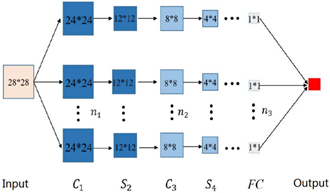Figure 1. The basic structure of a common DCNN

As shown in Figure 1, a 28*28 image is imported to the DCNN through matrix representation. Layer C1 is a convolutional layer that extracts the features from the input image. The n1 5*5 convolution kernels of layer C1 converts the input image into a 24*24 feature map. Layer S2 is a pooling layer with a 2*2 window. On this layer, the 24*24 feature map is down sampled into n1 12*12 feature maps. Layer C3 is another convolutional layer, which convolutes the n1 12*12 feature maps into n2 8*8 feature maps. Layer S4 is another pooling layer, which down samples the n2 8*8 feature maps to the size of 4*4. In the following layers, convolutional and pooling layers appear alternatively. On the final pooling layer, the activation value $\alpha$ is calculated layer by layer according to the network structure. This value is arranged in a column as per the vector, and the expanded vector serves as the input of the fully connected layer FC.

LeNet-5 model is a commonly used CNN . As shown in Figure 2, this lightweight DCNN contains all the basic components of the CNN in only eight layers.

## qqtu_pian_20201030141230.png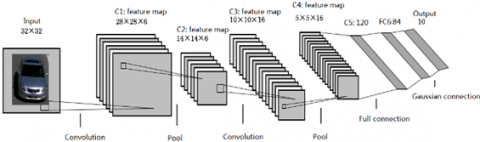Figure 2. The structure of LeNet-5

In Figure 2, the convolution represents the convolutional layer, which extracts features and reduces parameters; the pool represents the pooling layer, which samples the convoluted image to reduce the amount of data processing, preserving only the useful information.

The last layer of the CNN is often used to solve classification problems. Logical regression tools, such as softmax regression and SVM, can be used to classify the input image into two or more categories. Let {(x(1), y(1)), (x(2), y(2)),…,(x(w), y(w))} be the training set of w labeled images, where x(i) and y(i)∈{0,1} are the coordinates of image pixels.

The hypothesis function can be defined as:

$h_{9}=1 / 1+\exp \left(-\vartheta^{T} x\right)$     (1)

By training the model parameter $\vartheta$, the cost function can be minimized as:

$f(\vartheta)=-1 / w\left[\sum_{i=1}^{w} y^{\{i\}} \log h_{\vartheta}\left(x^{\{i\}}\right)\right.$$\left.\quad+\left(1-y^{\{i\}}\right) \log \left(1-h_{\vartheta}\left(x^{\{i\}}\right)\right)\right] (2) Many cases of vehicle recognition are multi-classification problems, which are beyond the capability of the traditional logical regression. Let {(x(1), y(1)), (x(2)), y(2)),…,(x(w), y(w))} be the training set, with y(i)∈{1,2,..,k}, and p(y=j|x) be the probability for training image x to fall into a class. Then, the hypothesis function h_{\vartheta}\left(x^{(i)}\right) can be defined as: h_{\vartheta}\left(x^{(i)}\right)=\left[\begin{array}{c}p\left(y^{(i)}=1 \mid x^{(i)} ; \vartheta\right) \\ p\left(y^{(i)}=2 \mid x^{(i)} ; \vartheta\right) \\ \ldots \\ p\left(y^{(i)}=k \mid x^{(i)} ; \vartheta\right)\end{array}\right] (3) The cost function of softmax classifier can be expressed as: f(\vartheta)=-1 / w\left[\sum_{i=1}^{w} \sum_{j=1}^{k} 1\left\{y^{(i)}=j\right\} \log e^{\vartheta_{j}^{T} x^{(i)}} / \sum_{s=1}^{k} e^{\vartheta_{l}^{T} x^{(i)}}\right] (4) where, 1{∙} is the indicator function. If the value of the expression is true, 1{∙} equals 1; otherwise, 1{∙} equals 0. During the softmax regression, an attenuation term is often added to the cost function, making the latter a strictly convex function: f(\vartheta)=-1 / w\left[\sum_{i=1}^{w} \sum_{j=1}^{k} 1\left\{y^{(i\}}=j\right\} \log e^{\vartheta_{j}^{T} x^{(i)}} / \sum_{s=1}^{k} e^{\vartheta_{l}^{T} x^{(i)}}\right]+$$\gamma / 2 \sum_{i=1}^{k} \sum_{j=0}^{n} \vartheta_{i j}^{2}$     (5)

This prevent local convergence in the optimization process.

3.2 Workflow of vehicle recognition algorithm

The basic flow of the vehicle recognition algorithm is shown in Figure 3.

First, the vehicle image to be recognized is selected from the original database, and imported to the vehicle recognition algorithm. Then, the target vehicle is detected in the original image by vehicle location method. After that, the vehicle type is recognized from the vehicle image.

In this paper, the vehicle dataset is collected from the vehicle image library of the HD Mount System in a city. The original images are in JPG format. With great differences in shooting environment, time, and angle, these images well reflect the actual recognition situation, providing good test samples for the vehicle recognition algorithm.

As shown in Figure 4, the original images often contain many things other than the target vehicle, such as pedestrians, other vehicles, and various other things. To ensure the recognition accuracy, the target vehicle should be located in each original image, before importing the image to the DCNN.

Here, the you look only once (YOLO) algorithm  is employed for target detection, because the algorithm can predict the positions of multiple targets and classify them all at once. Figure 5 provides an example image with vehicles located by YOLO.

## 2.png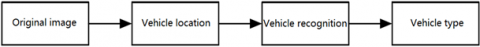Figure 3. The basic flow of the vehicle recognition algorithm

## 3.png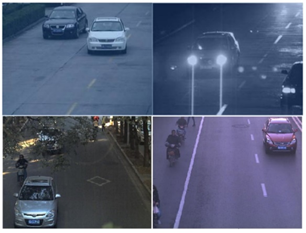Figure 4. The examples of vehicle dataset

## 4.png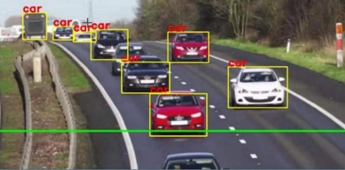Figure 5. The example of target vehicle location

3.3 DCNN-based vehicle recognition algorithm

In traditional image recognition, the features are generally extracted manually. The manual extraction may be effective on some objects. But it is far from ideal if there are various objects in the original images. Therefore, a vehicle recognition algorithm was proposed based on the DL (Figure 6).

## 5.pngFigure 6. The block diagram of the DCNN-based vehicle recognition algorithm

During the design of the DCNN, the following parameters were given special consideration: the size of input images, the number of output nodes, the number of convolution kernels, the kernel size, the activation function, and the network depth (i.e. the number of layers in the network).

The CNN requires the input images to have uniform size. However, the images after vehicle location are of different sizes. Therefore, these images were normalized into the same size of 256×256.

In this paper, vehicle of more than ten brands are labeled manually, and further divided by the model year. In this way, a total of 164 sub-models were obtained, creating a training set containing 164 types of vehicle images for the input layer of the DCNN. Therefore, the number of output nodes of the DCNN was set to 164.

In the output layer, the probability $p_{j}^{(i)}$ for the vehicle image x(i) to fall into class j is calculated by softmax classifier:

$p_{j}^{(i)}=\frac{\exp \left(W_{j}^{T} x^{(i)}+b_{j}\right)}{\sum_{j=0}^{163} \exp \left(W_{j}^{T} x^{(i)}+b_{j}\right)}$     (6)

Each feature map in the DCNN learns a feature of the input image. The number of feature maps equals the number of kernels. Therefore, the number of features depends on the number of kernels, which should be designed reasonably. If there are too few kernels, the extracted features will lack diversity; then, the network will learn a limited amount of information, and make inaccurate classifications. If there are too many kernels, the extracted features will be redundant, causing overfitting in network training; then, the training will consume too much time.

Therefore, the DCNNs with different number of kernels were trained and tested on the vehicle database. The relevant results are recorded in Table 1.

Table 1. The results on DCNNs with different number of kernels

 Number of kernels Accuracy on test set Accuracy on training set 32-64-128-128-256 0.95 0.88 64-128-256-265-384 0.91 0.85 128-256-384-384-384 0.97 0.91

The kernel size should adapt to the actual samples. In theory, the kernel size has a negative impact on the effect of feature extraction. Nevertheless, excessively small kernels cannot extract valid features, owing to the noise interference in images.

To determine the best kernel size, the DCNNs with kernel sizes of 5×5, 7×7, 9×9, and 11×11 were trained and tested on the vehicle database. The relevant results are recorded in Table 2.

Table 2. The results on DCNNs with different kernel sizes

 Kernel size Accuracy on test set Accuracy on training set 5×5 0.969 0.987 7×7 0.972 0.956 9×9 0.967 0.980 11×11 0.966 0.972

As shown in Table 2, the best accuracy was achieved at the kernel size of 7×7. With this kernel size, the response of the first convolutional layer can be observed through the visualization program. The weight diagram of the filter of the first convolution layer is shown in Figure 7.

## 6.png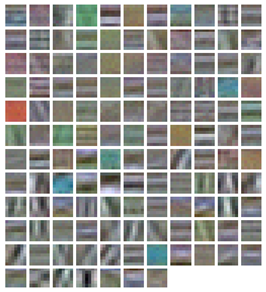Figure 7. The weight diagram of the first convolutional layer

The activation function has a great influence on the convergence of the CNN. Selecting a befitting activation function can speed up the convergence of the network. In our DCNN, rectified linear unit (ReLU) is adopted as the activation function . As shown in Figure 8, ReLU is a nonlinear unsaturated function that only needs a threshold to get the activation value:

$g(x)=\max (0, x)$     (7)

## 7.png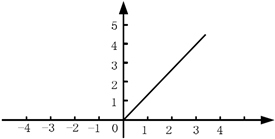Figure 8. Relu function

As shown in Figure 7, the output value of the function equals zero, if the calculated value is smaller than zero; the output value remains as it is, if the calculated value is greater than zero. This means ReLU as the activation function can accelerate the convergence and shorten the training time.

The network depth (i.e. the number of layers in the network) needs to match with the data volume. Considering the relatively small data size of the selected dataset, a simple DCNN structure was designed by comparing the training and testing results of four DCNNs with 9, 10, 11, and 12 layers, respectively, on the vehicle dataset. The relevant results are recorded in Table 3.

As shown in Table 3, with the increase of network depth, the accuracy on test set continued to improve. Of course, more network layers mean more network parameters, and greater computational complexity of the network.

Overall, the designed DCNN consists of 12 layers, including 6 convolutional layers, 3 pooling layers, 2 fully connected layers, and one softmax layer. On the last layer, softmax regression was adopted to classify the vehicles into different types.

Table 3. The results on DCNNs with different number of layers

 Network depth Accuracy on test set 9 0.922 10 0.956 11 0.962 12 0.966
4. Experiments and Result Analysis

The proposed DCNN was implemented under the DL framework of Caffe, using NVIDIA’s GK110 graphics processing unit (GPU). Step learning and momentum learning were selected for the training process, with the initial learning rate of 0.012 and the momentum of 0.85. After adjusting the number of iterations and training parameters, the mean test accuracy was 96.83% (Figure 9).

## 8.png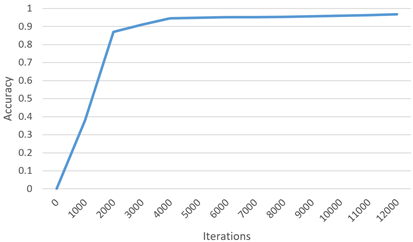Figure 9. The mean test accuracy

Then, several vehicle sub-models were randomly chosen from the test set. 100 images of each sub-model were selected to test the accuracy of our algorithm. The results on different sub-models are recorded in Table 4.

Table 4. The results on different sub-models

 Sub-model Number of recognition errors Accuracy on test set Audi A4L 3 0.97 BMW 5 Series 0 1 Benz E300 4 0.96 Peugeot 308 0 1 VW Polo 3 0.97 Toyota Camry 2 0.98 Nissan Teana 2 0.98 Mazda 6 3 0.97 Great Wall H6 0 1 Toyota Corolla 1 0.99

The experimental results show that the DCNN achieved a good recognition effect on the selected sub-models. During parameter adjustment, the training error rate and test error rate exhibited a downward trend, when number of iterations was fewer than 2,000, while the test accuracy showed an upward trend. This indicates that the network is in the learning state, and the iteration times can be increased to stabilize the accuracy. Of course, the number of iterations should not be too high. Otherwise, the training process will be too long.

In the training set, the images are distributed unevenly across sub-models. Some sub-models have thousands of images, while some have fewer than 100 images. Thus, the DCNN was tested on sub-models with a few samples, and those with many samples. The test results are recorded in Tables 5 and 6, respectively.

Table 5. The accuracy on sub-models with a few samples

 Sub-model Number of samples Number of testing samples Number of recognition errors Test accuracy Great Wall M4 96 24 1 0.958 Chevrolet Lova 88 22 0 1 Mazda CX5 92 23 0 1 Peugeot 508 87 21 1 0.952

Table 6. The accuracy on sub-models with many samples

 Sub-model Number of samples Number of testing samples Number of recognition errors Test accuracy VW Maogtan 2631 600 5 0.991 Citroen Elysee 1886 450 3 0.993 VW Bora 1530 400 10 0.975 Hyundai Elantra 1436 350 8 0.977

As shown in Tables 5 and 6, the accuracy of each sub-model was not directly proportional to the number of training samples in that sub-model. That is, the accuracy on sub-models with many training samples was not necessarily better, and that on sub-models with a few training samples was not necessarily worse. Of course, each sub-model must have enough number of training samples, allowing the DL to learn the vehicle features and continuous optimize network parameters. If there are too few images on a sub-model, the DCNN parameters cannot be optimized through continuous iteration.

5. Conclusions

The DL theory has been widely implemented in many fields. This paper designs a novel vehicle recognition algorithm based on the DL technology of DCNN. The proposed algorithm can extract vehicle features from the original images, continuously optimize the network parameters through feature learning, and classify the images with the softmax classifier. Experiments show that the proposed algorithm achieved an accuracy as high as 96.8%. The future research will further improve the training data, accuracy, and speed of the DCNN in vehicle recognition.

Acknowledgements

This work was supported by Key Project of Chinese Academy of Engineering under the subproject “Protection Policy of the Scarce Coking Coal Resources in China and Planning for the Coordinated Development between Coking Coal, Coke, and Steel Industries” (Grant No.: 2017-XZ-23), “Cultivation and Promotion of Protective Mining, Washing, and Dressing Technologies of Coking Coal” (Grant No.: 2017-XZ-24).

References

 Zhang, W., Qi, Q., Deng, J. (2017). Building intelligent transportation cloud data center based on SOA. International Journal of Ambient Computing and Intelligence (IJACI), 8(2): 1-11. https://doi.org/10.4018/IJACI.2017040101

 Olusanya, G.S., Eze, M.O., Ebiesuwa, O., Okunbor, C. (2020). Smart transportation system for solving urban traffic congestion. Review of Computer Engineering Studies, 7(3): 55-59. https://doi.org/10.18280/rces.070302

 Gregor, D., Toral, S., Ariza, T., Barrero, F., Gregor, R., Rodas, J., Arzamendia, M. (2016). A methodology for structured ontology construction applied to intelligent transportation systems. Computer Standards & Interfaces, 47: 108-119. https://doi.org/10.1016/j.csi.2015.10.002

 Pawłowicz, B., Trybus, B., Salach, M., Jankowski-Mihułowicz, P. (2020). Dynamic RFID identification in urban traffic management systems. Sensors, 20(15): 4225. https://doi.org/10.3390/s20154225

 Peng, J., Wang, H., Zhao, T., Fu, X. (2019). Learning multi-region features for vehicle re-identification with context-based ranking method. Neurocomputing, 359: 427-437. https://doi.org/10.1016/j.neucom.2019.06.013

 Hellinga, B., Knapp, G. (2000). Automatic vehicle identification technology-based freeway incident detection. Transportation Research Record, 1727(1): 142-153. https://doi.org/10.3141/1727-18

 Iwasaki, Y., Kawata, S., Nakamiya, T. (2011). Robust vehicle detection even in poor visibility conditions using infrared thermal images and its application to road traffic flow monitoring. Measurement Science and Technology, 22(8): 085501. https://doi.org/10.1088/0957-0233/22/8/085501

 Stiawan, R., Kusumadjati, A., Aminah, N.S., Djamal, M., Viridi, S. (2019). An ultrasonic sensor system for vehicle detection application. In Journal of Physics: Conference Series, 1204(1): 012017.

 Hinton, G.E., Salakhutdinov, R.R. (2006). Reducing the dimensionality of data with neural networks. Science, 313(5786): 504-507. https://doi.org/10.1126/science.1127647

 LeCun, Y., Bengio, Y., Hinton, G. (2015). Deep learning. Nature, 521(7553): 436-444.

 Chen, F.C., Jahanshahi, M.R. (2017). NB-CNN: Deep learning-based crack detection using convolutional neural network and Naïve Bayes data fusion. IEEE Transactions on Industrial Electronics, 65(5): 4392-4400. https://doi.org/10.1109/TIE.2017.2764844

 Ali, H., Tran, S.N., Benetos, E., Garcez, A.S.D.A. (2018). Speaker recognition with hybrid features from a deep belief network. Neural Computing and Applications, 29(6): 13-19. https://doi.org/10.1007/s00521-016-2501-7

 PÉGNY, M., Ibnouhsein, I. (2018). Quelle transparence pour les algorithmes d’apprentissage machine? Revue d’intelligence Artificielle, Revue d'Intelligence Artificielle, 32(4): 447-478. https://doi.org/10.3166/ria.32.447-478

 Duong, C.N., Luu, K., Quach, K.G., Bui, T.D. (2019). Deep appearance models: A deep Boltzmann machine approach for face modeling. International Journal of Computer Vision, 127(5): 437-455. https://doi.org/10.1007/s11263-018-1113-3

 Sun, G., Sheng, B., Dong, L. (2018). New trend of image recognition and feature extraction technology introduction. Traitement du Signal, 35: 3-4.

 Liu, R., Han, Q., Min, W., Zhou, L., Xu, J. (2019). Vehicle logo recognition based on enhanced matching for small objects, constrained region and SSFPD network. Sensors, 19(20), 4528. https://doi.org/10.3390/s19204528

 Yu, Y., Wang, J., Lu, J., Xie, Y., Nie, Z. (2018). Vehicle logo recognition based on overlapping enhanced patterns of oriented edge magnitudes. Computers & Electrical Engineering, 71: 273-283. https://doi.org/10.1016/j.compeleceng.2018.07.045

 Dong, Z., Wu, Y., Pei, M., Jia, Y. (2015). Vehicle type classification using a semisupervised convolutional neural network. IEEE Transactions on Intelligent Transportation Systems, 16(4): 2247-2256. https://doi.org/10.1109/TITS.2015.2402438

 Fu, H., Ma, H., Wang, G., Zhang, X., Zhang, Y. (2020). MCFF-CNN: Multiscale comprehensive feature fusion convolutional neural network for vehicle color recognition based on residual learning. Neurocomputing, 395: 178-187. https://doi.org/10.1016/j.neucom.2018.02.111

 Zhuang, X., Kang, W., Wu, Q. (2016). Real-time vehicle detection with foreground-based cascade classifier. IET Image Processing, 10(4): 289-296. https://doi.org/10.1049/iet-ipr.2015.0333

 Ma, Y.J., Ma, Y.T., Chen, J.H. (2019). Vehicle recognition based on multi-layer features of convolutional neural network and support vector machine. Laser & Optoelectronics Progress, 56(14): 141001.

 Choi, H.C., Park, J.M., Choi, W.S., Oh, S.Y. (2012). Vision-based fusion of robust lane tracking and forward vehicle detection in a real driving environment. International Journal of Automotive Technology, 13(4): 653-669. https://doi.org/10.1007%2Fs12239-012-0064-x

 Zhang, Y., Wang, J., Yang, X. (2017). Real-time vehicle detection and tracking in video based on faster R-CNN. In Journal of Physics: Conference Series, 887(1): 012068. https://doi.org/10.1088/1742-6596/887/1/012068

 Yu, S., Wu, Y., Li, W., Song, Z., Zeng, W. (2017). A model for fine-grained vehicle classification based on deep learning. Neurocomputing, 257: 97-103. https://doi.org/10.1016/j.neucom.2016.09.116

 Dooley, D., McGinley, B., Hughes, C., Kilmartin, L., Jones, E., Glavin, M. (2015). A blind-zone detection method using a rear-mounted fisheye camera with combination of vehicle detection methods. IEEE Transactions on Intelligent Transportation Systems, 17(1): 264-278. https://doi.org/10.1109/TITS.2015.2467357

 Arounachalam, V.V., Latchoumi, T.P., Bhavya, B., Sultana, S.S. (2019). Object detection in convolution neural networks using iterative refinements. Revue d'Intelligence Artificielle, 33(5): 367-372. https://doi.org/10.18280/ria.330506

 Han, J., Liao, Y., Zhang, J., Wang, S., Li, S. (2018). Target fusion detection of LiDAR and camera based on the improved YOLO algorithm. Mathematics, 6(10): 213. https://doi.org/10.3390/math6100213

 Sstla, V., Kolli, V.K.K., Voggu, L.K., Bhavanam, R., Vallabhasoyula, S. (2020). Predictive model for network intrusion detection system using deep learning. Revue d'Intelligence Artificielle, 34(3): 323-330. https://doi.org/10.18280/ria.340310